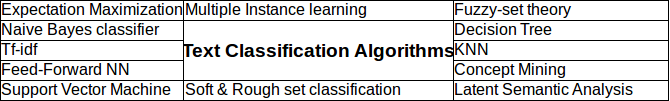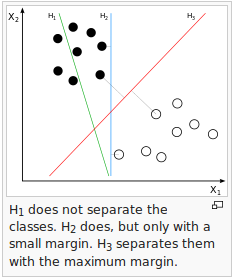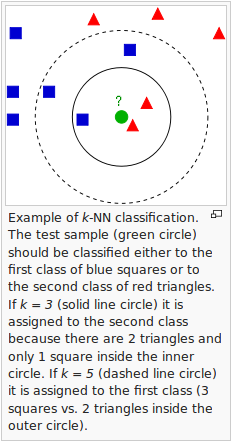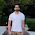## Wednesday, 24 August 2016

### Text Classification Algorithms

Document classification or text classification has been used in many areas like spam filtering, email routing, sentiment analysis, readability analysis. In recent years, it has become important for social media sites to understand the text.Here is a brief overview of different text classification algorithms.Decision Tree- Tree models where the target variable can take a finite set of values are called classification trees. In these tree structures, leaves represent class labels and branches represent interaction of features that lead to those class labels. Decision trees where the target variable can take continuous values are called regression trees.

Tf-idf -tf–idf-  It is short for term frequency–inverse document frequency, is a numerical statistic that is intended to reflect how important a word is to a document in a collection.Variations of the tf–idf weighting scheme are often used by search engines as a central tool in scoring and ranking a document's relevance given a user query.Naive Bayes classifier- It is based on the Bayes theorm hence it's a probabilistic model. Despite their naive design and apparently oversimplified assumptions, naive Bayes classifiers have worked quite well in many complex real-world situations. An advantage of naive Bayes is that it only requires a small amount of training data to estimate the parameters necessary for classification.

Support Vector machine- An SVM model is a representation of the examples as points in space, mapped so that the examples of the separate categories are divided by a clear gap that is as wide as possible. New examples are then mapped into that same space and predicted to belong to a category based on which side of the gap they fall on. Hence it is a non probabilistic model.

Neural Network- neural networks have been used to solve a wide variety of tasks, like computer vision and speech recognition that are hard to solve using ordinary rule based programming In machine learning, an artificial neural network (ANN) is a network inspired by biological neural networks (the central nervous system of animals, in particular the brain) which are used to estimate or approximate functions that can depend on a large number of inputs that are generally unknown.# p { margin-bottom: 0.25cm; line-height: 120%; }a:link { }

In k-NN classification- the output is a class membership. An object is classified by a majority vote of its neighbors, with the object being assigned to the class most common among its k nearest neighbors (k is a positive integer typically small). If k = 1, then the object is simply assigned to the class of that single nearest neighbor.)

Multiple-instance learning- It is a supervised learning where Instead of receiving a set of instances which are individually labeled, the learner receives a set of labeled bags, each containing many instances. In the simple case of multiple-instance binary classification, a bag may be labeled negative if all the instances in it are negative. On the other hand, a bag is labeled positive if there is at least one instance in it which is positive. From a collection of labeled bags, the learner tries to either (i) induce a concept that will label individual instances correctly or (ii) learn how to label bags without inducing the concept.

Fuzzy-set theory- It is based on fuzzy logic where the membership functions used to map predictors into fuzzy sets.

Concept Mining – Used to identify idea/concept of a document.It provides a powerful insights into the meaning, provenance and similarity of documents.

Latent semantic analysis (LSA)- It is a technique of analyzing relationships between a set of documents and the terms they contain by producing a set of concepts related to the documents and terms. LSA assumes that words that are close in meaning will occur in similar pieces of text. A matrix containing word counts per paragraph (rows represent unique words and columns represent each paragraph) is constructed from a large piece of text and a mathematical technique called singular value decomposition (SVD) is used to reduce the number of rows while preserving the similarity structure among columns. Words are then compared by taking the cosine of the angle between the two vectors formed by any two rows. Values close to 1 represent very similar words while values close to 0 represent very dissimilar words.

Expectation maximization algo- It is an iterative algorithm and based on maximum likelihood of parameters.

http://machinelearningstories.blogspot.in/2016_11_01_archive.html

read another blog to know relation between time series and simple regression analysis-

1.1.